# Rhombus MATH

Construct a rhombus M A T H with diagonal MT=4cm, angle MAT=120°

Result

m =  2.309 cm

#### Solution:Leave us a comment of example and its solution (i.e. if it is still somewhat unclear...):

Showing 1 comment:Solustion2
Constructions steps:

1. make angle XAY = 120 deg
2. draw its axis p througth point A....
3. draw two paralels lines l1,l2 to axis p at distance 4/2 = 2 cm
4. intersections of legs of angle XAY and l1, l2 is points is points M and T.
5. make point H - use symmetry of point A in mirror of line MTrhombus#### To solve this example are needed these knowledge from mathematics:

See also our right triangle calculator. See also our trigonometric triangle calculator.

## Next similar examples:

1. Construct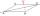Construct a rhombus ABCD, if the size of the diagonal AC is 6 cm and diagonal BD 8 cm long.
2. Rhombus constructionConstruct ABCD rhombus if its diagonal AC=9 cm and side AB = 6 cm. Inscribe a circle in it touching all sides...
3. Park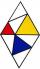In the park is marked diamond shaped line connecting locations A, D, S, C, B, A. Calculate its length if |AB| = 108 m, |AC| = 172.8 m.
4. Diagonals in diamons/rhombusRhombus ABCD has side length AB = 4 cm and a length of one diagonal of 6.4 cm. Calculate the length of the other diagonal.
5. Diamond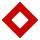Side length of diamond is 35 cm and the length of the diagonal is 56 cm. Calculate the height and length of the second diagonal.
6. Diagonal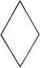Can be a diagonal of diamond twice longer than it side?
7. Ruler and compassUse a ruler and compass to construct a triangle ABC with AB 5cm BAC 60° and ACB 45°.
8. Triangle SSAConstruct a triangle ABC if |AB| = 5cm va = 3cm, CAB = 50 °. It is to create the analysis and construction steps.
9. Height 2Calculate the height of the equilateral triangle with side 38.
10. SatinSanusha buys a piece of satin 2.4 m wide. The diagonal length of the fabric is 4m. What is the length of the piece of satin?
11. 30-60-90The longer leg of a 30°-60°-90° triangle measures 5. What is the length of the shorter leg?
12. ClimbFor horizontal distance 4.9 km road rise by 6.1 m. Calculate the road pitch in ‰ (permille, parts per thousand).
13. Obtuse angle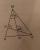The line OH is the height of the triangle DOM, line MN is the bisector of angle DMO. obtuse angle between the lines MN and OH is four times larger than the angle DMN. What size is the angle DMO? (see attached image)
14. Find the 9Find the missing angle in the triangle and then name triangle. Angles are: 95, 2x+15, x+3
15. Double ladderThe double ladder is 8.5m long. It is built so that its lower ends are 3.5 meters apart. How high does the upper end of the ladder reach?
16. Double ladderThe double ladder shoulders should be 3 meters long. What height will the upper top of the ladder reach if the lower ends are 1.8 meters apart?
17. Center traverseIt is true that the middle traverse bisects the triangle?